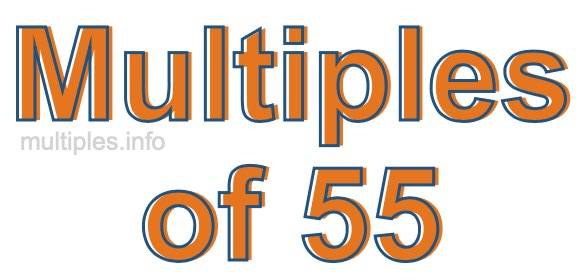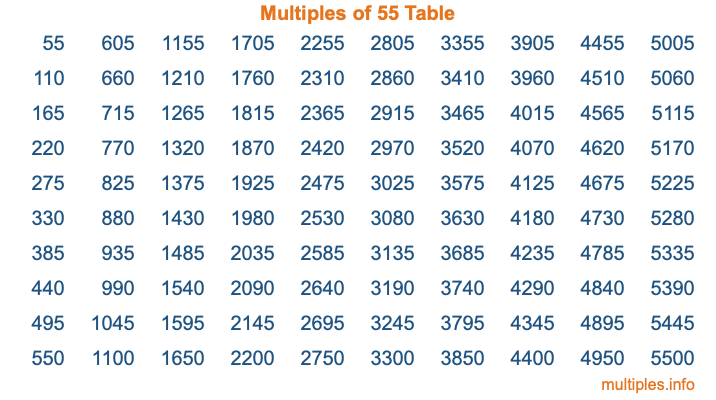Multiples of 55Welcome to the Multiples of 55 page. Here we will first teach you everything you will ever need to know about the multiples of 55, and then give you a study guide summary of everything we taught you to make sure you remember it all. Use this page to look up facts and learn information about the multiples of 55. This page will make you a multiples of fifty-five expert!

Definition of Multiples of 55
Multiples of 55 are all the numbers that when divided by 55 equal an integer. Each of the multiples of 55 are called a multiple. A multiple of 55 is created by multiplying 55 by an integer.

Therefore, to create a list of multiples of 55, you start with 1 multiplied by 55, then 2 multiplied by 55, then 3 multiplied by 55, and so on for as long as you want. Thus, the list of the first five multiples of 55 is 55, 110, 165, 220, and 275. To see a larger list of multiples of 55, see the printable image of Multiples of 55 further down on this page. We also have a category where you can choose any nth multiple of 55.

Multiples of 55 Checker
The Multiples of 55 Checker below checks to see if any number of your choice is a multiple of 55. In other words, it checks to see if there is any number (integer) that when multiplied by 55 will equal your number. To do that, we divide your number by 55. If the the quotient is an integer, then your number is a multiple of 55.

Is  a multiple of 55?

Least Common Multiple of 55 and ...
A Least Common Multiple (LCM) is the lowest multiple that two or more numbers have in common. This is also called the smallest common multiple or lowest common multiple and is useful to know when you are adding our subtracting fractions. Enter one or more numbers below (55 is already entered) to find the LCM.

Check out our LCM Calculator if you need more details about the Least Common Multiple or if you need the LCM for different numbers for adding and subtraction fractions.

nth Multiple of 55
As we stated above, 55 is the first multiple of 55, 110 is the second multiple of 55, 165 is the third multiple of 55, and so on. Enter a number below to find the nth multiple of 55.

th multiple of 55

Multiples of 55 vs Factors of 55
55 is a multiple of 55 and a factor of 55, but that is where the similarities end. All postive multiples of 55 are 55 or greater than 55. All positive factors of 55 are 55 or less than 55.

Below is the beginning list of multiples of 55 and the factors of 55 so you can compare:

Multiples of 55: 55, 110, 165, 220, 275, etc.

Factors of 55: 1, 5, 11, 55

As you can see, the multiples of 55 are all the numbers that you can divide by 55 to get a whole number. The factors of 55, on the other hand, are all the whole numbers that you can multiply by another whole number to get 55.

It's also interesting to note that if a number (x) is a factor of 55, then 55 will also be a multiple of that number (x).

Multiples of 55 vs Divisors of 55
The divisors of 55 are all the integers that 55 can be divided by evenly. Below is a list of the divisors of 55.

Divisors of 55: 1, 5, 11, 55

The interesting thing to note here is that if you take any multiple of 55 and divide it by a divisor of 55, you will see that the quotient is an integer.

Multiples of 55 Table
Below is an image of the first 100 multiples of 55 in a table. The table is in chronological order, column by column. The first column has the first ten multiples of 55, the second column has the next ten multiples of 55, and so on.The Multiples of 55 Table is also referred to as the 55 Times Table or Times Table of 55. You are welcome to print out our table for your studies.

Negative Multiples of 55
Although not often discussed or needed in math, it is worth mentioning that you can make a list of negative multiples of 55 by multiplying 55 by -1, then by -2, then by -3, and so on, to get the following list of negative multiples of 55:

-55, -110, -165, -220, -275, etc.

Multiples of 55 Summary
Below is a summary of important Multiples of 55 facts that we have discussed on this page. To retain the knowledge on this page, we recommend that you read through the summary and explain to yourself or a study partner why they hold true.

There are an infinite number of multiples of 55.

A multiple of 55 divided by 55 will equal a whole number.

55 divided by a factor of 55 equals a divisor of 55.

The nth multiple of 55 is n times 55.

The largest factor of 55 is equal to the first positive multiple of 55.

55 is a multiple of every factor of 55.

55 is a multiple of 55.

A multiple of 55 divided by a divisor of 55 equals an integer.

55 divided by a divisor of 55 equals a factor of 55.

Any integer times 55 will equal a multiple of 55.

Multiples of a Number
Here you can get the multiples of another number, all with the same attention to detail as we did for multiples of 55 on this page.

Multiples of
Multiples of 56
Did you find our page about multiples of fifty-five educational? Do you want more knowledge? Check out the multiples of the next number on our list!